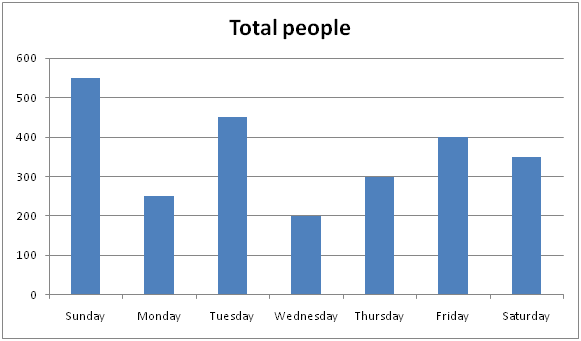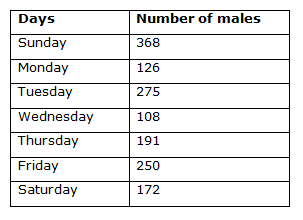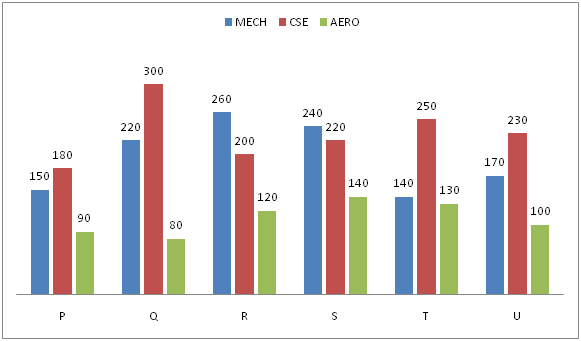# Quantitative Aptitude Questions (Data Interpretation) for IBPS RRB Assistant Prelims 2018 Day-167

Dear Readers, IBPS RRB is conducting Online Examination for the recruitment of clerical cadre To enrich your preparation here we have providing new series of Data Interpretation – Quantitative Aptitude Questions. Candidates those who are appearing in IBPS RRB Assistant Prelims Exams can practice these Quantitative Aptitude average questions daily and make your preparation effective.

[WpProQuiz 3236]

Click “Start Quiz” to attend these Questions and view Solutions

Directions (1 – 5): Study the following graph carefully and answer the given questions:

Following bar graph shows total number of people went for a movie in different days of a week in a certain village and the table shows the total number of males among them.1) Total number of females went for a movie in Tuesday and Friday together is approximately what percentage of total number of males went for a movie in Monday and Wednesday together?

a) 120 %

b) 105 %

c) 140 %

d) 160 %

e) 90 %

2) Find the ratio between the total number of males went for a movie in Sunday and Saturday together to that of total number of females went for a movie in Thursday and Wednesday together?

a) 180: 67

b) 152: 53

c) 217: 93

d) 375: 158

e) None of these

3) If 34 % of total number of people went for a movie in Sunday bought AC tickets, then find the difference between total number of people buy AC tickets in Sunday to that of total number of females went for a movie in Monday?

a) 82

b) 86

c) 75

d) 63

e) None of these

4) In which of the following days, the lowest number of females went for a movie?

a) Thursday

b) Wednesday

c) Monday

d) Tuesday

e) None of these

5) Find the average number of males went for a movie in Monday to Friday?

a) 210

b) 170

c) 190

d) 180

e) None of these

Directions (6 – 10): Study the following graph carefully and answer the given questions:

Following bar graph shows the total number of students studying different streams in various engineering colleges.6) Find the difference between the total number of students studying in College Q to that in College T of all the given streams?

a) 80

b) 120

c) 110

d) 180

e) None of these

7) Find the ratio between the total number of students studying CSE in college P, R and T together to that of total number of students studying AERO in college Q, S and U together?

a) 52: 17

b) 46: 15

c) 38: 21

d) 63: 32

e) None of these

8) Total number of MECH students studying in college P, Q and R together is what percentage of total number of students studying in college S together?

a) 85 %

b) 120 %

c) 105 %

d) 135 %

e) None of these

9) Find the total number of students in college R, T and U together in all the given streams?

a) 1800

b) 1600

c) 1540

d) 1480

e) None of these

10) Find the total number of AERO students studying in all the given colleges together?

a) 660

b) 780

c) 550

d) 820

e) None of these

Total number of females went for a movie in Tuesday and Friday together

= > (450 – 275) + (400 – 250)

= > 175 + 150 = 325

Total number of males went for a movie in Monday and Wednesday together

= > 126 + 108 = 234

Required % = (325/234)*100 = 138. 8 % = 140 %

The total number of males went for a movie in Sunday and Saturday together

= > 368 + 172 = 540

The total number of females went for a movie in Thursday and Wednesday together

= > (300 – 191) + (200 – 108)

= > 109 + 92 = 201

Required ratio = 540: 201 = 180: 67

34 % of total number of people went for a movie in Sunday bought AC tickets

= > 550*(34/100) = 187

Total number of females went for a movie in Monday

= > 250 – 126 = 124

Required difference = 187 – 124 = 63

In Sunday = > 550 – 368 = 182

In Monday = > 250 – 126 = 124

In Tuesday = > 450 – 275 =175

In Wednesday = > 200 – 108 = 92

In Thursday = > 300 – 191 = 109

In Friday = > 400 – 250 = 150

In Saturday = > 350 – 172 = 178

The lowest number of females went for a movie in Wednesday.

The average number of males went for a movie in Monday to Friday

= > (126 + 275 + 108 + 191 + 250)/5

= > 950/5 = 190

The total number of students studying in College Q of all the given streams

= > 220 + 300 + 80 = 600

The total number of students studying in College S of all the given streams

= > 140 + 250 + 130 = 520

Required difference = 600 – 520 = 80

The total number of students studying CSE in college P, R and T together

= > 180 + 200 + 250 = 630

The total number of students studying AERO in college Q, S and U together

= > 80 + 140 + 100 = 320

Required ratio = 630: 320 = 63: 32

Total number of MECH students studying in college P, Q and R together

= > 150 + 220 + 260 = 630

Total number of students studying in college S together

= > 240 + 220 + 140 = 600

Required % = (630/600)*100 = 105 %

The total number of students in college R, T and U together in all the given streams

= > (260 + 200 + 120) + (140 + 250 + 130) + (170 + 230 + 100)

= > 580 + 520 + 500 = 1600

The total number of AERO students studying in all the given colleges together

= > 90 + 80 + 120 + 140 + 130 + 100

= > 660

Daily Practice Test Schedule | Good Luck

 Topic Daily Publishing Time Daily News Papers & Editorials 8.00 AM Current Affairs Quiz 9.00 AM Quantitative Aptitude “20-20” 11.00 AM Vocabulary (Based on The Hindu) 12.00 PM General Awareness “20-20” 1.00 PM English Language “20-20” 2.00 PM Reasoning Puzzles & Seating 4.00 PM Daily Current Affairs Updates 5.00 PM Data Interpretation / Application Sums (Topic Wise) 6.00 PM Reasoning Ability “20-20” 7.00 PM English Language (New Pattern Questions) 8.00 PM